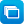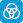# Mathematics / Year 10 / Statistics and Probability / Data representation and interpretation

Curriculum content descriptions

Use scatter plots to investigate and comment on relationships between two numerical variables (ACMSP251)

Elaborations
• using authentic data to construct scatter plots, make comparisons and draw conclusions
General capabilities
• Literacy Literacy
• Numeracy Numeracy
• Critical and creative thinking Critical and creative thinking
ScOT terms

Data analysis,  Scatter plots

## Refine results by

Year level 9-10
Resource type
Learning area Mathematics

## Refine by topic

Related topic### A mathematical focus on 'Choose your own statistics'

This teaching resource demonstrates ways in which real data can be interpreted and analysed. It is based on the interactive learning resource Choose your own statistics, in which data is presented as infographics, graphical representations and tables. The focus of the supporting teaching and learning activities is on analysing ...### Choose your own statistics

Explore the way in which statistics are presented using a wide range of graphs types for ten topics related to human rights. Customise and publish graphs and download data to a spreadsheet program such as Excel to enable further exploration.### Gapminder world

This is an interactive resource featuring an animated scatter plot displaying the development of over 200 countries between 1800 and 2011 based on data for two variables, the default being income per person and life expectancy, with population size represented by the size of the plotted points (bubbles). The graph has a ...### Secondary mathematics: using real data

These seven learning activities, which focus on the use of 'real data' using a variety of tools (software) and devices (hardware), illustrate the ways in which content, pedagogy and technology can be successfully and effectively integrated in order to promote learning. In the activities, teachers use the three content strands ...### Graph investigator

Explore different graph types such as scatter plots, line graphs and histograms. Choose questions to investigate. For example, do girls have faster reaction times than boys? Select the appropriate data set. Then choose a useful graph type. Examine the data presented in the chosen graph type and draw a conclusion about the ...### Graph investigator: types of graphs

This learning object shows a variety of graphs used in data handling and explains their features, their different uses and their advantages. Graph types include stem-and-leaf plots, histogram, box-and-whisker, scatter plot, pie graph, line graph and column graph. This learning object is one in a series of seven objects. ...### Scatter plots

Find out what a scatter plot is and how it can be used to investigate relationships between two variables. Analyse data for a pair of variables such as hand span and foot length. Enter your personal data and plot it with other data on a graph. Use a line of best fit to identify if there is a positive or negative relationship ...### Scatter plots: foot length and hand span

Find out what a scatter plot is and how it can be used to investigate relationships between two variables. Analyse data for a pair of variables: hand span and foot length. Enter your personal data and plot it with other data on a graph. Use a line of best fit to identify if there is a positive or negative relationship between ...### Graphing: line of best fit

Plot some data points on a graph. Estimate the position for a line of best fit. Use a graphing tool to check your estimate. Add more points to see how they affect the position of the line of best fit.### Teacher's guide: global development quiz

This is a teacher resource with a downloadable guide describing a number of quizzes based on Gapminder world for use with a computer and projector in a whole-class situation. Each quiz is based on an animated scatter plot and requires students to compare the development of countries between 1800 and 2011 based on data for ...### Scatter plots: create your own data

Find out what a scatter plot is and how it can be used to investigate relationships between two variables. Gather and analyse data for a pair of variables such as hand span and foot length. Enter your data set and plot it on a graph. Use a line of best fit to identify if there is a positive or negative relationship between ...### Data representation and interpretation using scatterplots - teacher resource

This resource is a professional learning package designed for pre-service teachers. It demonstrates and investigates the interrelated content, pedagogy and technology knowledge used in a series of mathematics lessons that focus on looking for a relationship between two variables. The resource provides video footage of a ...### Analysing data using scatter plots

This is a collection of 18 digital curriculum resources that focus on how scatter plots are drawn and used to analyse the relationship between two continuous variables. The collection is organised into five sections: what this type of graph is used for; looking more closely at scatter plots; using scatter plots to describe ...### Scatter plots: age and reaction time

Find out what a scatter plot is and how it can be used to investigate relationships between two variables. Analyse data for a pair of variables: a person's age and the time it takes them to react to a signal. Enter your personal data and plot it with other data on a graph. Use a line of best fit to identify if there is ...### Scatter plots: height and bellybutton height

Find out what a scatter plot is and how it can be used to investigate relationships between two variables. Analyse data for a pair of variables: a person's height and the height of their bellybutton measured from the floor. Enter your personal data and plot it with other data on a graph. Use a line of best fit to identify ...### Teacher's guide: 200 years that changed the world

This is a teacher resource explaining how to use an animated scatter plot from Gapminder world in the context of a presentation to students about global development over the past 200 years. The resource consists of a step-by-step guide to making effective use of the scatter plot, which represents the development in wealth ...### Data in Gapminder world

This resource is a dataset providing data for a number of indicators of development over time for more than 200 countries. The indicators fall into the following categories: population, health, economy, environment, work, infrastructure, energy and education, with over 500 sets of data in total. Each set of data can be ...### Scatter plots: about scatter plots

Find out what a scatter plot is and how it can be used to investigate relationships between two variables. Examine data for a pair of variables such as a person's height at the age of 2 and their height at the age of 22. Look at the data plotted on a graph. Notice how a line of best fit is used to identify if there is a ...### Scatter plots: age and reaction time [ESL]

Find out what a scatter plot is and how it can be used to investigate relationships between two variables. Analyse data for a pair of variables: a person's age and the time it takes them to react to a signal. Enter your personal data and plot it with other data on a graph. Use a line of best fit to identify if there is ...### Scatter plots: create your own data [ESL]

Find out what a scatter plot is and how it can be used to investigate relationships between two variables. Gather and analyse data for a pair of variables such as hand span and foot length. Enter your data set and plot it on a graph. Use a line of best fit to identify if there is a positive or negative relationship between ...